# Stacked Attention Networks for Image Question Answering

## WHY?

Visual question answering task is answering natural language questions based on images. To solve questions that require multi-step reasoning, stacked attention networks(SANs) stacks several layers of attention on parts of images based on query.

## WHAT?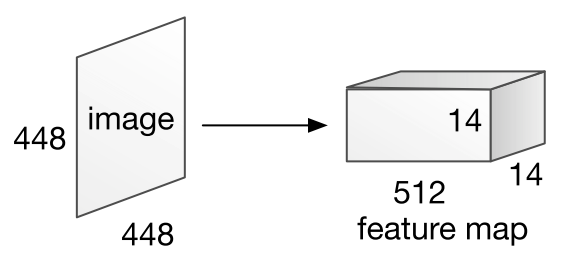Image model extracts feature map from image with VGGNet structure.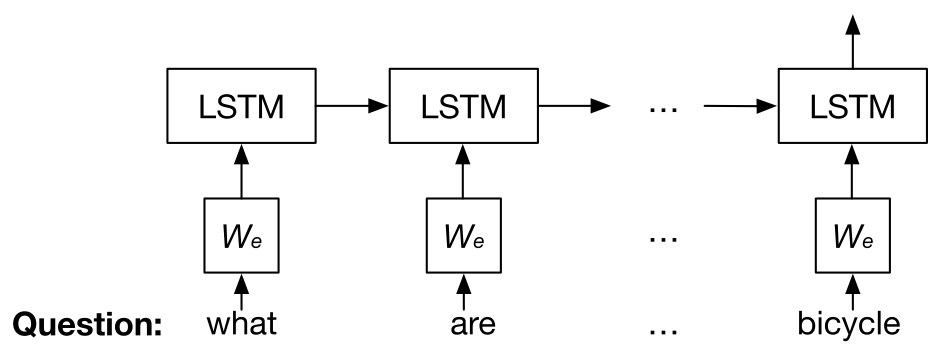Question model uses the final layer of LSTM to encode question.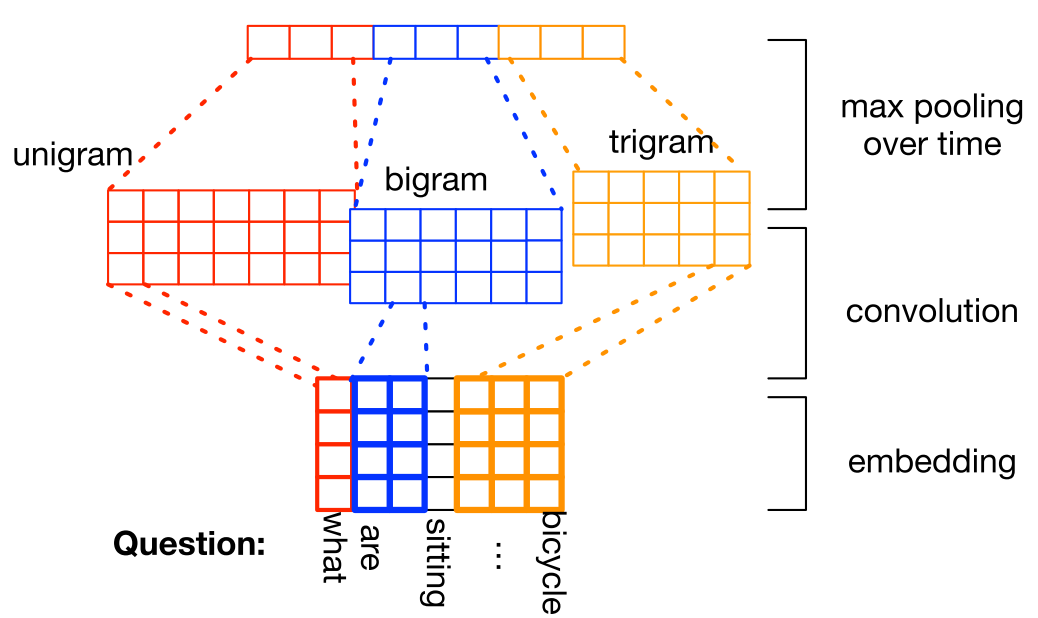Question can also be encoded through CNN based question model.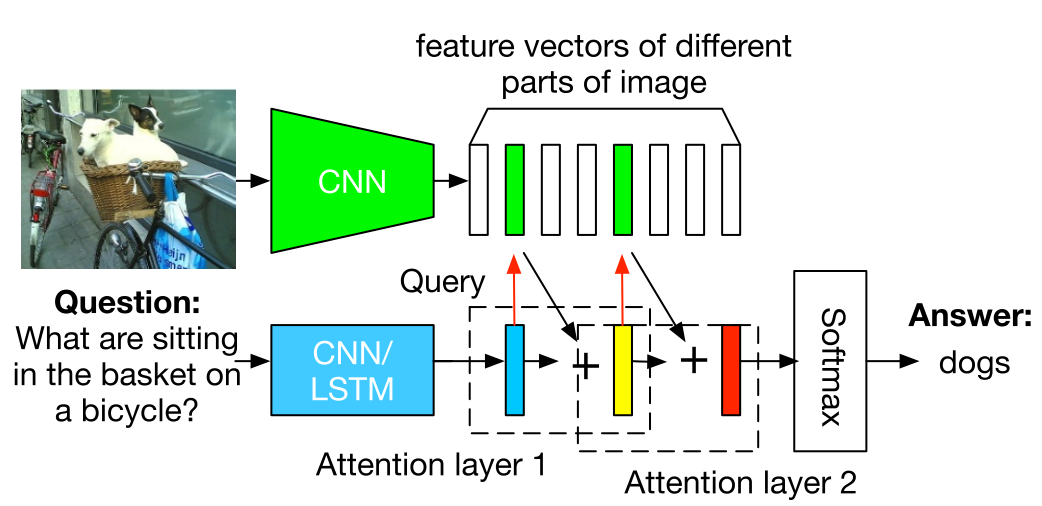Using the extracted features of images(v_I$v_I$) and texts(v_Q$v_Q$), attention is applied to image. Several layers of attentions can be stacked to progressively pay attention.

h_A = tanh(W_{I,A}v_I\oplus(W_{Q,A}v_Q + b_A))\\
p_I = softmax(W_P h_A + b_P)\\
\tilde{v}_I = \sum_i p_i v_i\\
u = \tilde{v}_I + v_Q\\

h_A^k = tanh(W^k_{I,A}v_I\oplus(W^k_{Q,A}u^{k-1} + b^k_A))\\
p^k_I = softmax(W^k_P h^k_A + b^k_P)\\
\tilde{v}^k_I = \sum_i p^k_i v_i\\
u^k = \tilde{v}^k_I + u^{k-1}\\
p_{ans} = softmax(W_u u^K + b_u)

## So?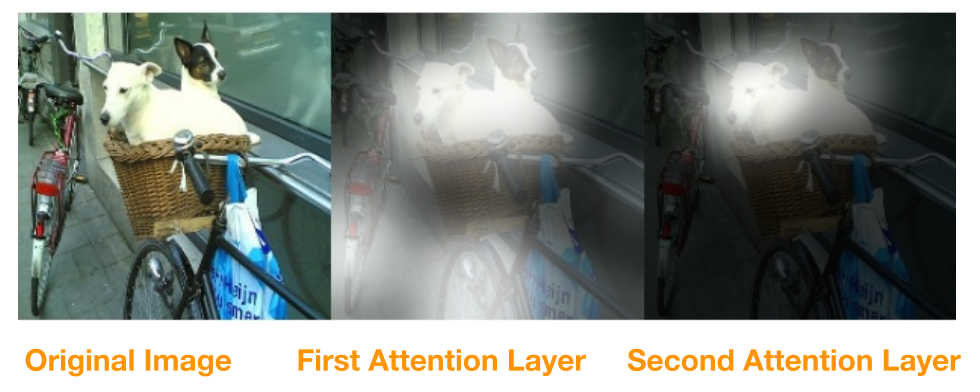SAN achieved SOTA results in DAQUAR-ALL, DAQUAR-REDUCED, COCO-QA and VQA. Also, the learned layers of attention showed progressive focusing of important part of image.

## Critic

Fundamental paper in VQA area.

Powered by aiden